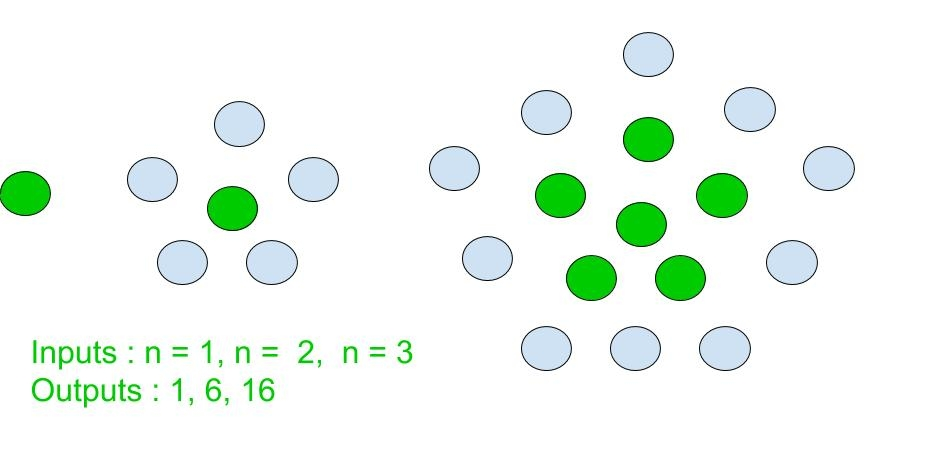# Centered pentagonal number

Given an integer n, find the nth Centered pentagonal number.

A Centered Pentagonal Number is a centered figurate number that represents a pentagon with a dot in the centre and other dots surrounding it in pentagonal layers successively [ Source: Wiki ]Few Centred pentagonal Number are :
1, 6, 16, 31, 51, 76, 106, 141, 181, 226, 276, 331, 391………………..

Examples :

```Input : 3
Output : 16

Input : 9
Output : 181
```

## Recommended: Please try your approach on {IDE} first, before moving on to the solution.

Approach:
Centered pentagonal for n-th term is given by :

``````

Basic implementation of the above approach :

## C++

 `// Program to find nth ` `// Centered pentagonal number. ` `#include ` `using` `namespace` `std; ` ` `  `// centered pentagonal number function ` ` `  `int` `centered_pentagonal_Num(``int` `n) ` `{ ` `    ``// Formula to calculate nth ` `    ``// Centered pentagonal number ` `    ``// and return it into main function. ` ` `  `    ``return` `(5 * n * n - 5 * n + 2) / 2; ` `} ` ` `  `// Driver Code ` `int` `main() ` `{ ` `    ``int` `n = 7; ` `    ``cout << n << ``"th Centered pentagonal number: "``; ` `    ``cout << centered_pentagonal_Num(n); ` `    ``return` `0; ` `} `

## Java

 `// Program to find nth ` `// Centered pentagonal number ` `import` `java.io.*; ` ` `  `class` `GFG  ` `{ ` `     `  `// centered pentagonal ` `// number function ` `static` `int` `centered_pentagonal_Num(``int` `n) ` `{ ` `    ``// Formula to calculate  ` `    ``// nth Centered pentagonal  ` `    ``// number and return it  ` `    ``// into main function. ` ` `  `    ``return` `(``5` `* n * n - ``5` `* n + ``2``) / ``2``; ` `} ` ` `  `// Driver Code ` `public` `static` `void` `main (String[] args)  ` `{ ` `int` `n = ``7``; ` `System.out.print(n + ``"th Centered "` `+  ` `              ``"pentagonal number: "``); ` `System.out.println(centered_pentagonal_Num(n)); ` `} ` `} ` ` `  `// This code is contributed by anuj_67. `

## Python3

 `# Python program to find Nth ` `# Centered pentagonal number. ` ` `  `# Function to calculate ` `# Centered pentagonal number. ` ` `  `def` `centered_pentagonal_Num(n): ` ` `  `    ``# Formula to calculate nth ` `    ``# Centered pentagonal number. ` `     `  `    ``return` `(``5` `*` `n ``*` `n ``-` `5` `*` `n ``+` `2``) ``/``/` `2` ` `  `# Driver Code ` `n ``=` `7` `print``(``"%sth Centered pentagonal number : "` `%``n, ` `                    ``centered_pentagonal_Num(n)) ` `                     `  `# This code is contributed by ajit                  `

## C#

 `// C# Program to find nth ` `// Centered pentagonal number ` `using` `System; ` ` `  `class` `GFG  ` `{ ` `     `  `// centered pentagonal ` `// number function ` `static` `int` `centered_pentagonal_Num(``int` `n) ` `{ ` `    ``// Formula to calculate  ` `    ``// nth Centered pentagonal  ` `    ``// number and return it  ` `    ``// into main function. ` ` `  `    ``return` `(5 * n * n - 5 * n + 2) / 2; ` `} ` ` `  `// Driver Code ` `public` `static` `void` `Main ()  ` `{ ` `int` `n = 7; ` `Console.Write(n + ``"th Centered "` `+  ` `           ``"pentagonal number: "``); ` `Console.WriteLine(centered_pentagonal_Num(n)); ` `} ` `} ` ` `  `// This code is contributed by anuj_67. `

## PHP

 ` `

Output :

```7th Centered pentagonal number: 106
```

My Personal Notes arrow_drop_upCheck out this Author's contributed articles.

If you like GeeksforGeeks and would like to contribute, you can also write an article using contribute.geeksforgeeks.org or mail your article to contribute@geeksforgeeks.org. See your article appearing on the GeeksforGeeks main page and help other Geeks.

Please Improve this article if you find anything incorrect by clicking on the "Improve Article" button below.

Improved By : jit_t, vt_m

Article Tags :
Practice Tags :

Be the First to upvote.

Please write to us at contribute@geeksforgeeks.org to report any issue with the above content.